﻿ 长江上游梯级水电站群的水能宏观关系研究

# 长江上游梯级水电站群的水能宏观关系研究Relationship between Water and Energy for Cascade Hydropower Stations in Upper Reaches of the Yangtze River

Abstract: Hydropower stations convert water energy into electricity. The estimation of hydropower generation of power stations and water resources of basins from each other can be directly implemented in a simple way, which provides a basis for water-energy conversion. The 21 large-scale cascade hydropower stations in upper reaches of the Yangtze River were selected as the case study, and the conventional and deterministic optimization operations were used to simulate reservoir operation, and then the water-energy conversion relationship was established. The results show that: 1) the linear relationship between the outflow and power generation is significant for a single hydropower station; 2) there is a linear relationship between the total water resources of the basin and the total power generation of the cascade hydropower stations and 3) the water energy relationship has a better goodness of fitting when the installed capacity is used as the weight. The proposed method provides a new way to estimate the amount of water resources based on power generation, and to estimate the power generation from water resources.

1. 引言

2. 研究方法

2.1. 水–能关系构建

2.1.1. 单个水电站

$E=N\cdot t=k\cdot q\cdot h\cdot t$ (1)

$E=k\left({q}_{0}\cdot {h}_{0}+{h}_{0}\left(q-{q}_{0}\right)+{q}_{0}\left(h-{h}_{0}\right)+HOT\right)\cdot t$ (2)

$E=k\left({h}_{0}q+{q}_{0}h-{q}_{0}{h}_{0}\right)\cdot t$ (3)

$E=\left(a\cdot q+b\right)\cdot t$ (4)

2.1.2. 梯级水电站

${E}_{总}={N}_{总}\cdot t=\left(A\cdot Q+B\right)\cdot t$ (5)

2.2. 梯级水电站群调度模型

2.2.1. 目标函数

$E=\mathrm{max}\underset{k=1}{\overset{M}{\sum }}{E}_{i}$ (6)

2.2.2. 约束条件

1) 水量平衡约束：

${V}_{i+1,j}={V}_{i,j}+\left({I}_{i,j}-{Q}_{i,j}\right)\cdot t$ (7)

2) 水电站库容约束：

$V{L}_{i,j}\le {V}_{i,j}\le V{U}_{i,j}$ (8)

3) 水电站出库流量约束：

$Q{L}_{i,j}\le {Q}_{i,j}\le Q{U}_{i,j}$ (9)

4) 水电站出力约束：

$P{L}_{i,j}\le {P}_{i,j}\le P{U}_{i,j}$ (10)

5) 非负约束及其他约束。

2.2.3. 模型求解

3. 案例分析

3.1. 长江上游梯级水电站群调度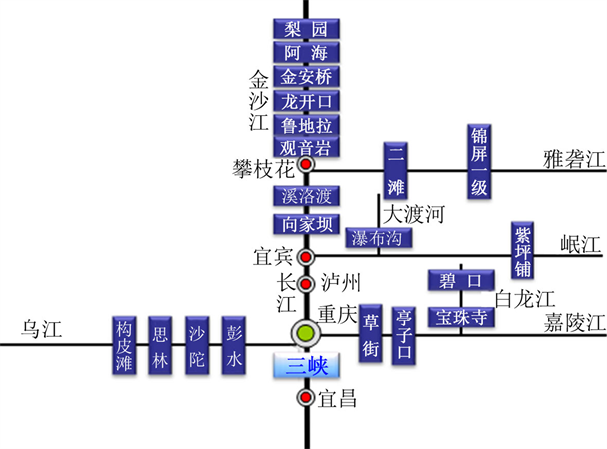Figure 1. Overview of hydropower stations in the upper reaches of the Yangtze RiverTable 1. The results of cascade hydropower station group

3.2. 推求水–能转化关系

3.2.1. 单个水电站

1) 以水定电Table 2. The power generation and water resources fitting results of the Three Gorges hydropower station

Table 3. The water-energy relationship fitting results of a single hydropower station

2) 以电定水

3.2.2. 梯级水电站

1) 直接拟合

1、以水定电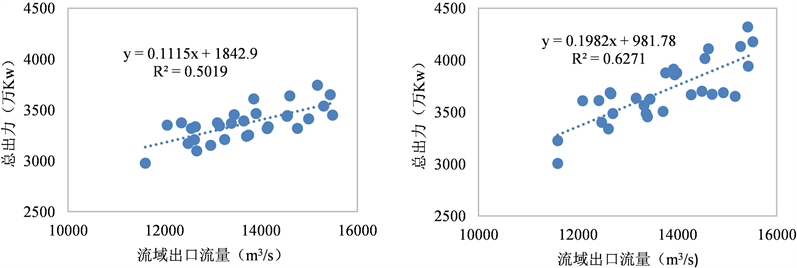(a) 常规调度水–能关系 (b) 优化调度水–能关系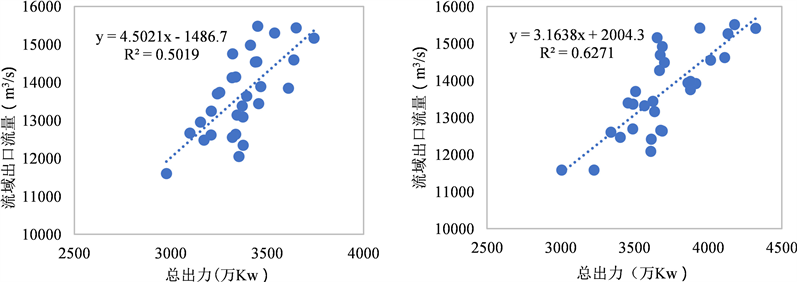(c) 常规调度能–水关系 (d) 优化调度能–水关系

Figure 2. The energy-water relationship calibration results of the hydropower station groupTable 4. The power generation and water resources fitting result of the hydropower station group

2、以电定水

2) 权重拟合

4. 结论及展望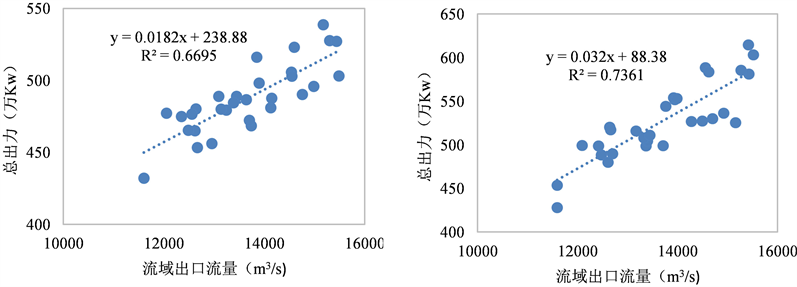(a) 常规调度水–能关系 (b) 优化调度水–能关系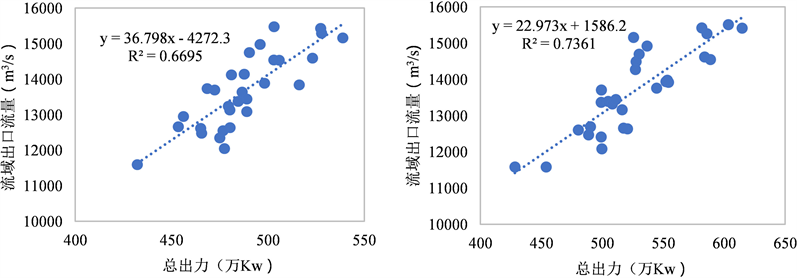(c) 常规调度能–水关系 (d) 优化调度能–水关系

Figure 3. The weighted water-energy relationship calibration results of the hydropower station group

1) 推求单个水电站的特性参数，建立适用于各水电站的水–能转化关系；

2) 基于聚合思想，可构建各水电站电量累加值与流域水资源总量的宏观年尺度水–能转化关系；以各电站装机容量作为权重，推求的水–能转化关系拟合效果更佳。

3) 以水–能转化关系为基础，可通过流域实际发电量推求流域水资源量。

1) 部分水库单库的拟合效果不佳，应进一步分析原因并考虑其他优选的拟合方式。

2) 可以考虑更多的水库权重确定方式，并加以比较，以确定最佳的权重确定方法，综合考虑流域内各水电站的影响。

NOTES

*通讯作者。

 MING, B., LIU, P., GUO, S., et al. Optimizing utility-scale photovoltaic power generation for integration into a hydropower reservoir by incorporating long- and short-term operational decisions. Applied Energy, 2017, 204: 432-445.
https://doi.org/10.1016/j.apenergy.2017.07.046

 陈进. 长江流域水资源调控与水库群调度[J]. 水利学报, 2018, 49(1): 2-8. CHEN Jin. Regulation of water resources and operation of reservoir group in the Yangtze River basin. Journal of Hydraulic Engineering, 2018, 49(1): 2-8. (in Chinese)

 FAN, J.-L., et al. Relationship between energy production and water resource utilization: A panel data analysis of 31 provinces in China. Journal of Cleaner Production, 2017, 167: 88-96.
https://doi.org/10.1016/j.jclepro.2017.08.167

 贾东旭. 提高水电站水库水能利用率的方法[J]. 水电自动化与大坝监测, 2002, 26(2): 54-58, 64. JIA Dongxu. Method of improving water power utilization rate and hydropower stations. Hydropower Automation and Dam Monitoring, 2002, 26(2): 54-58, 64. (in Chinese)

 刘心愿, 郭生练, 刘攀, 李响. 考虑综合利用要求的三峡水库提前蓄水方案[J]. 水科学进展, 2009, 20(6): 851-856. LIU Xinyuan, GUO Shenglian, LIU Pan and LI Xiang. Scheme of impounding in advance for the Three Gorges Reservoir by considering the comprehensive utilization benefits. Advances in Water Science, 2009, 20(6): 851-856. (in Chinese)

 姚磊. 水轮发电机增容改造技术对水轮机提高水能利用率的影响[J]. 电力系统装备, 2017(11): 77, 135. YAO Lei. Influence of hydraulic turbine generator capacity increasing technology on hydraulic energy utilization rate of hydraulic turbine. Electric System, 2017(11): 77, 135. (in Chinese)

 TSOLAS, S. D., KARIM, M. N. and HASAN, M. M. F. Optimization of water-energy nexus: A network representation-based graphical approach. Applied Energy, 2018, 224: 230-250.
https://doi.org/10.1016/j.apenergy.2018.04.094

 JU-HWAN, Y. Maximization of hydropower generation through the application of a linear programming model. Journal of Hydrology, 2009, 376(1-2): 182-187.
https://doi.org/10.1016/j.jhydrol.2009.07.026

 陈西臻, 刘攀, 何素明, 张旺, 李泽君, 张靖文. 基于聚合-分解的并联水库群防洪优化调度研究[J]. 水资源研究, 2015(4): 23-31. CHEN Xizhen, LIU Pan, HE Suming, ZHANG Wang, LI Zejun and ZHANG Jinwen. Optimization of parallel reservoirs for flood control based on the aggregation-decomposition method. Journal of Water Resources Research, 2015(4): 23-31. (in Chinese)
https://doi.org/10.12677/JWRR.2015.41003

 马立亚, 雷晓辉, 蒋云钟, 王浩. 基于DPSA的梯级水库群优化调度[J]. 中国水利水电科学研究院学报, 2012, 10(2): 140-145. MA Liya, LEI Xiaohui, JIANG Yunzhong and WANG Hao. Optimal operation of cascade reservoirs based on DPSA. Journal of China Institute of Water Resources and Hydropower Research, 2012, 10(2): 140-145. (in Chinese)

 李克飞, 武见, 董滇红, 王林威. 基于DPSA的水库优化调度方案研究[J]. 人民黄河, 2015, 37(10): 128-130. LI Kefei, WU Jian, DONG Dianhong and WANG Linwei. Research on reservoir optimization scheduling scheme based on DPSA. Yellow River, 2015, 37(10): 128-130. (in Chinese)

 郭生练, 陈炯宏, 刘攀. 水库群联合优化调度研究进展与展望[J]. 水科学进展, 2010, 21(4): 496-503. GUO Shenglian, CHEN Jionghong, LIU Pan. State-of-the-art review of joint operation for multi-reservoir systems. Advances in Water Science, 2010, 21(4): 496-503. (in Chinese)

 刘丹雅. 三峡及长江上游水库群水资源综合利用调度研究[J]. 人民长江, 2010, 41(15): 5-9. LIU Danya. Study on water resources comprehensive utilization scheduling of the Three Gorges and the upper reaches of the Yangtze River. Yangtze River, 2010, 41(15): 5-9. (in Chinese)

 丁毅, 傅巧萍. 长江上游梯级水库群蓄水方式初步研究[J]. 人民长江, 2013, 44(10): 72-75. DING Yi, FU Qiaoping. Preliminary study on water storage method of cascade reservoirs in the upper reaches of the Yangtze River. Yangtze River, 2013, 44(10): 72-75. (in Chinese).

Top